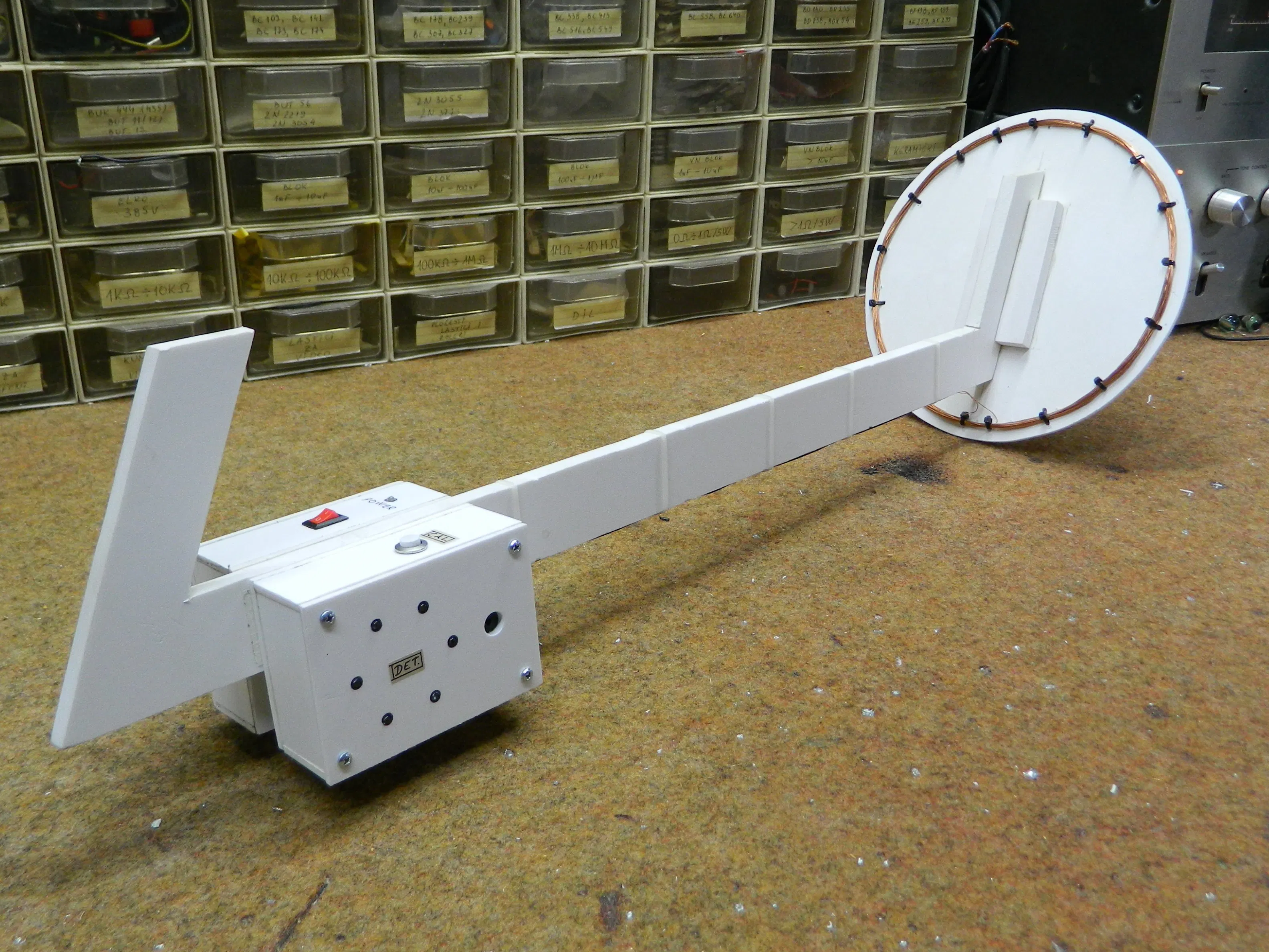Arduino nano metal detector project by Mirko Pavleski - Printable Version +- Computer Aided Automation (https://computeraidedautomation.com) +-- Forum: CAA Discussions (https://computeraidedautomation.com/forum-7.html) +--- Forum: Robotics and Microcontrollers (https://computeraidedautomation.com/forum-8.html) +---- Forum: Arduinos (https://computeraidedautomation.com/forum-9.html) +---- Thread: Arduino nano metal detector project by Mirko Pavleski (/thread-142.html) Arduino nano metal detector project by Mirko Pavleski - techberth - 27-12-2020 Interesting Arduino nano metal detector project by Mirko PavleskiArduino nano - code Code:```// PI metal detector for arduino version_18_min (C) alex --- 1967 2015 int ss0 = 0; int ss1 = 0; int ss2 = 0; long c0 = 0; long c1 = 0; long c2 = 0; byte i = 0; int sss0 = 0; int sss1 = 0; int sss2 = 0; int s0 = 0; int s1 = 0; int s2 = 0; void setup () { DDRB = 0xFF; // port B - all out DDRD = 0xFF; // port D - all out for (i = 0; i <255; i ++) // calibration / calibration { PORTB = B11111111; // translate the pins of port B to 1, thus opening. key (turn on) delayMicroseconds (200); // wait 200 microseconds PORTB = 0; // delayMicroseconds (20); s0 = analogRead (A0); s1 = analogRead (A0); s2 = analogRead (A0); c0 = c0 + s0; c1 = c1 + s1; c2 = c2 + s2; delay (3); } c0 = c0 / 255; c0 = c0 - 5; c1 = c1 / 255; c1 = c1 - 5; c2 = c2 / 255; c2 = c2 - 5; } void loop () { PORTB = B11111111; // translate the pins of port B to 1, thus opening. key (turn on) delayMicroseconds (200); // wait 200 microseconds PORTB = 0; // close the key by interrupting the current in the search coil (turn off - interrupt circut in search coil) delayMicroseconds (20); s0 = analogRead (A0); s1 = analogRead (A0); s2 = analogRead (A0); ss0 = s0 - c0; if (ss0 <0) { sss0 = 1; } ss0 = ss0 / 16; PORTD = ss0; // send to the indicator (send to LEDs) delay (1); ss1 = s1 - c1; if (ss1 <0) { sss1 = 1; } ss1 = ss1 / 16; PORTD = ss1; // send to the indicator (send to LEDs) delay (1); ss2 = s2 - c2; if (ss2 <0) { sss2 = 1; } ss2 = ss2 / 16; PORTD = ss2; // send to the indicator (send to LEDs) delay (1); if (sss0 + sss1 + sss2> 2) { digitalWrite (7, HIGH); digitalWrite (6, HIGH); digitalWrite (5, HIGH); digitalWrite (4, HIGH); digitalWrite (3, HIGH); digitalWrite (2, HIGH); digitalWrite (1, HIGH); digitalWrite (0, HIGH); delay (1); sss0 = 0; sss1 = 0; sss2 = 0; } }``` circuit diagram : Nano Metal Detector DIY Project source : https://create.arduino.cc/projecthub/mircemk/diy-simple-sensitive-metal-detector-7f34ad?ref=platform&ref_id=424_trending___&offset=0RE: Arduino nano metal detector project by Mirko Pavleski - orlando - 28-03-2021 nice project thanks for sharing arduino metal detector project